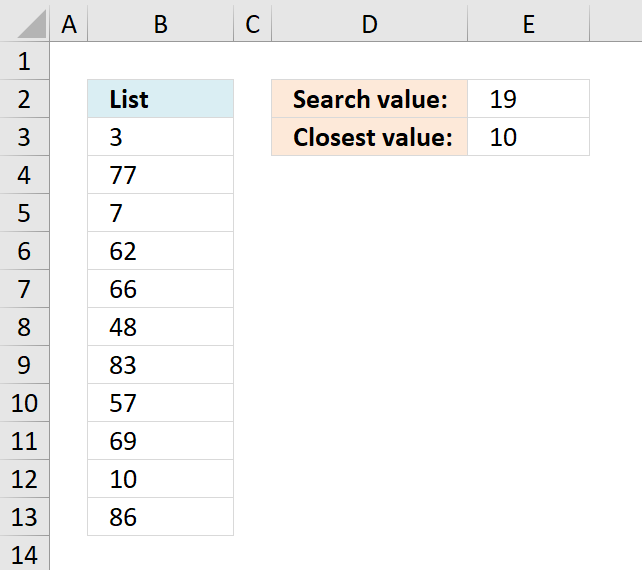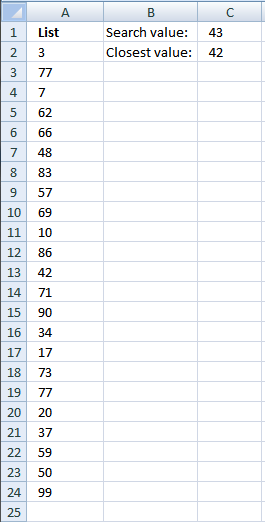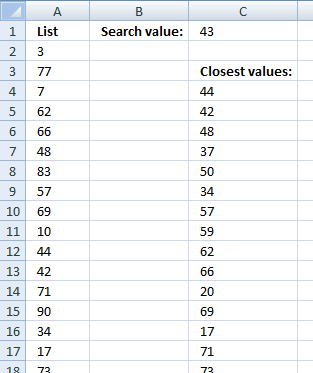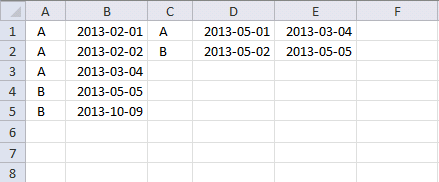Author: Oscar Cronquist Article last updated on December 27, 2018### Find closest value

Question: How to find closest number in a list?Array formula in cell C2:

=INDEX(A2:A26,MATCH(MIN(ABS(A2:A26-C1)),ABS(A2:A26-C1),0))

Recommended article

Find latest date based on a condition

How to create an array formula

1. Copy (Ctrl + c) and paste (Ctrl + v) array formula into formula bar.
2. Press and hold Ctrl + Shift.
3. Press Enter once
4. Release all keys

Recommended article

How to enter an array formula

Array formulas allows you to do advanced calculations not possible with regular formulas.

Explaining array formula

INDEX(A2:A26,MATCH(MIN(ABS(A2:A26-C1)),ABS(A2:A26-C1),0))

Step 1 - Return absolute values of numbers in an array, numbers without its sign

INDEX(A2:A26,MATCH(MIN(ABS(A2:A26-C1)),ABS(A2:A26-C1),0))

ABS(number) returns the absolute value of a number, a number without its sign.

ABS(A2:A26-C1)

becomes

ABS({3, 77, 7, 62, 66, 48, 83, 57, 69, 10, 86, 42, 71, 90, 34, 17, 73, 77, 20, 37, 59, 50, 99} - 43)

becomes

ABS{-40, 34, -36, 19, 23, 5, 40, 14, 26, -33, 43, -1, 28, 47, -9, -26, 30, 34, -23, -6, 16, 7, 56})

and returns

{40, 34, 36, 19, 23, 5, 40, 14, 26, 33, 43, 1, 28, 47, 9, 26, 30, 34, 23, 6, 16, 7, 56}

Step 2 - Return the smallest number

INDEX(A2:A26,MATCH(MIN(ABS(A2:A26-C1)),ABS(A2:A26-C1),0))

MIN(number1,[number2]) returns the smallest number in a set of values. Ignores logical values and text

MIN(ABS(A2:A26-C1))

MIN({40, 34, 36, 19, 23, 5, 40, 14, 26, 33, 43, 1, 28, 47, 9, 26, 30, 34, 23, 6, 16, 7, 56}

returns 1.

Step 3 - Return the relative position of an item in an array

INDEX(A2:A26,MATCH(MIN(ABS(A2:A26-C1)),ABS(A2:A26-C1),0))

MATCH(lookup_value;lookup_array; [match_type]) returns the relative position of an item in an array that matches a specified value

MATCH(MIN(ABS(A2:A26-C1)),ABS(A2:A26-C1),0)

becomes

MATCH(1, {40, 34, 36, 19, 23, 5, 40, 14, 26, 33, 43, 1, 28, 47, 9, 26, 30, 34, 23, 6, 16, 7, 56}, 0)

returns 12.

Step 4 - Return a value or reference of the cell at the intersection of a particular row and column

INDEX(array,row_num,[column_num]) returns a value or reference of the cell at the intersection of a particular row and column, in a given range

INDEX(A2:A26,MATCH(MIN(ABS(A2:A26-C1)),ABS(A2:A26-C1),0))

becomes

INDEX(A2:A26,12)

and returns 42.

EDIT: Added excel workbook to this blog postfind-closest-value.xls

### How to find closest valuesArray formula in cell C4:

=INDEX(\$A\$2:\$A\$24, MATCH(SMALL(ABS(\$A\$2:\$A\$24-\$E\$1), ROW(A1)), IF(COUNTIF(\$C\$3:C3, \$A\$2:\$A\$24)<COUNTIF(\$A\$2:\$A\$24, \$A\$2:\$A\$24), ABS(\$A\$2:\$A\$24-\$E\$1), "A"), 0))

How to create an array formula

1. Select cell C4
2. Copy (Ctrl + c) and paste (Ctrl + v) array formula into formula bar.
3. Press and hold Ctrl + Shift.
4. Press Enter once
5. Release all keys

How to copy array formula

1. Select cell C4
2. Copy cell c4 (Ctrl + c)
3. Select cell range C4:C20
4. Paste (Ctrl + v)

find-closest-values.xlsfind-closest-values1.xls

### How to find closest values and return adjacent valuesArray formula in cell D5:

=INDEX(\$A\$2:\$A\$24, MATCH(SMALL(ABS(\$A\$2:\$A\$24-\$E\$1), ROW(A1)), IF(COUNTIF(\$D\$4:D4, \$A\$2:\$A\$24)<COUNTIF(\$A\$2:\$A\$24, \$A\$2:\$A\$24), ABS(\$A\$2:\$A\$24-\$E\$1), "A"), 0))

Array formula in cell E5:

=INDEX(\$B\$2:\$B\$24, MIN(IF((D5=\$A\$2:\$A\$24)*(COUNTIFS(\$D\$4:D4, \$A\$2:\$A\$24, \$E\$4:E4, \$B\$2:\$B\$24)<COUNTIFS(\$A\$2:\$A\$24, \$A\$2:\$A\$24, \$B\$2:\$B\$24, \$B\$2:\$B\$24)), MATCH(ROW(\$A\$2:\$A\$24), ROW(\$A\$2:\$A\$24)), "A")))

How to create an array formula

1. Select cell D5
2. Copy (Ctrl + c) and paste (Ctrl + v) array formula into formula bar.
3. Press and hold Ctrl + Shift.
4. Press Enter once
5. Release all keys

How to copy array formula

1. Select cell D5
2. Copy (Ctrl + c)
3. Select cell range D6:D28
4. Paste (Ctrl + v)

### Find closest value with criterion

Basically I have two columns of data containing the same entries so assume column A and column C are two such columns.and column B and column D have certain dates corresponding to these entries.I need a formulae which will first match the entries in column A wih entries in column C .then compare the date in column D with the dates in column B and then throw up the nearest date.So my data sheet looks something like this:
Col A Col B Col C Col D Col E(result)
A 1/2/2013 A 5/1/2013 4/3/2013
A 2/2/2013 B 5/2/2013 5/5/2013
A 4/3/2013
B 5/5/2013
B 9/10/2013 As you can see the formulae first compares the entries in col C (A) with the entries in col A (all the A's) then it matches the date in col D (5/1/2013) with the dates pertaining to value A in col B.The closest date then is 4/3/2013 which is the answer.Array formula in cell E1:

=INDEX(\$B\$1:\$B\$5,MATCH(MIN(ABS(IF(C1=\$A\$1:\$A\$5,\$B\$1:\$B\$5-D1,9.99999999999999E+307))),ABS(IF(C1=\$A\$1:\$A\$5,\$B\$1:\$B\$5-D1,9.99999999999999E+307)),0))

Workbook Find closest value